Present value (PV) is the current value of a future cash flow, given a specific rate of return. Analysts and investors are able to account for the time value of money, which states that an amount of money today is worth more than that same amount in the future (due to its future earning potential).

Present value is useful for determining how much money must be invested today in order to be a certain amount in the future. It’s also an effective tool for stock and bond pricing, banking, financial modeling, and more.

You can absolutely calculate PV on your own, but it’s much easier to find present value in Excel or a financial calculator – which we teach you here.

## How to Calculate Present Value in Excel

Excel’s auto feature automatically calculates various functions and recalculates every time a value, formula, or name is changed. Once the PV function has been entered, the auto feature allows you to calculate present value – simply by entering the appropriate values.

### The CPT PV Formula in Excel

In order to calculate present value in Excel, you’ll need to use the CPT PV formula:

= PV(rate, nper, pmt, [fv], [type])

Where:

PV: Present Value
Rate: Interest rate per payment period
Nper: Number of payment periods
Pmt: Payment per period (amount, including principal and interest)
Fv: Future value. If left blank, value is assumed to be 0
Type: When payments are due (beginning or end of period)

## Calculating PV in Excel Step-by-Step

Now that we’ve established the CPT PV formula and the required information, you can calculate present value in Excel.

Let’s say you want to reach a savings goal of \$25,000 in 10 years and your savings account pays 5% interest per year. In this situation, you’d use the following inputs to find out how much you would need to put into the account today in order to meet your savings goal:

PV: leave blank
Rate: 5%
Nper: 10
Pmt: \$0 (because we are not making payments)
Fv: \$25,000

### Create a Table

Start by creating a table to organize your information.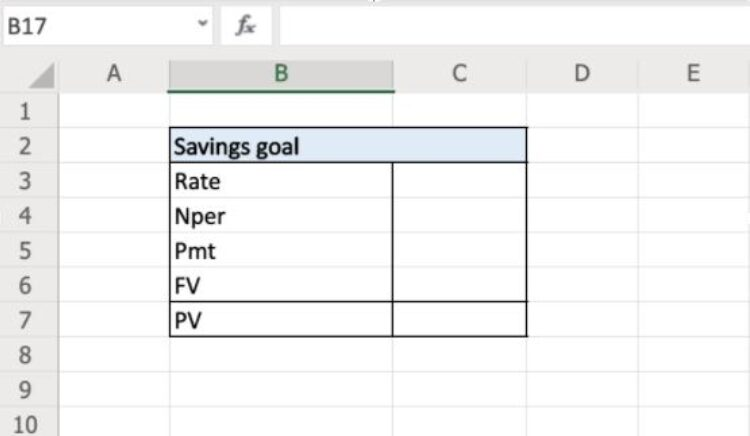Next, you’ll enter the required information from above. While you can add things like dollar and percent signs, they are not necessary: Excel can handle the calculation with or without them.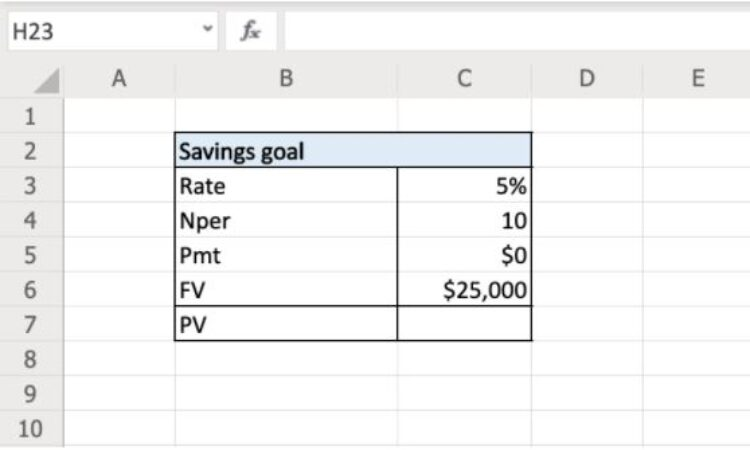### Enter the Present Value Formula

Enter the present value formula. Click the blank cell to the right of your desired calculation (in this case, C7) and enter the PV formula: = PV(rate, nper, pmt, [fv]).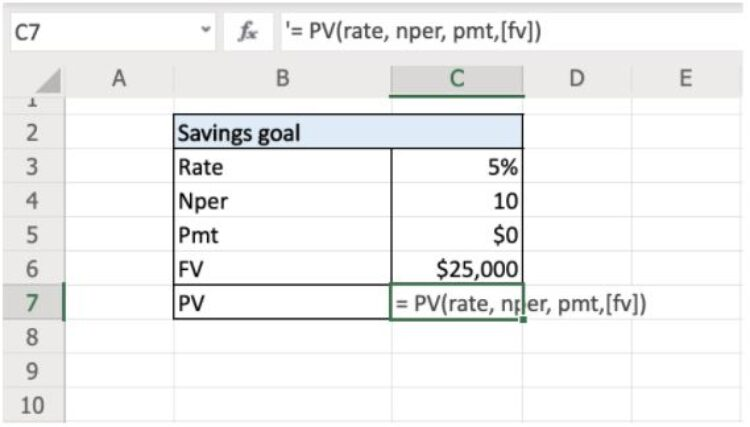Note: The calculation will not work yet. You will need to follow through with the next step in order to calculate the present value based on your inputs.

### Select The Corresponding Cells

Change the values in the PV formula (rate, nper, pmt, [fv]) to the cells that correspond with your information. In this situation, it is cells c3,c4,c5, and c6.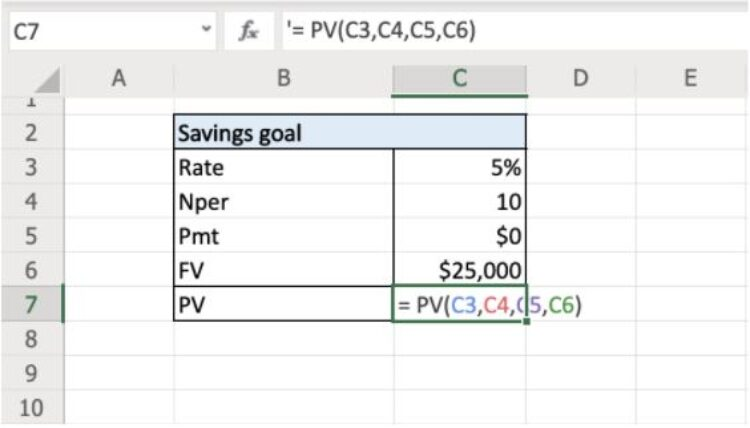Once you’ve selected the relevant cells, hit ‘enter’. The cell will now show the amount that you must put into the account today in order for it to grow to \$25,000 in 10 years.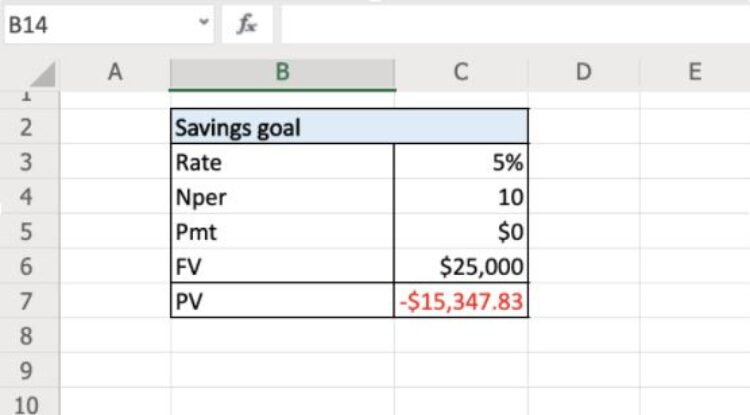Note: The present value will be negative because it is considered a cash outflow.

## How to Use a Financial Calculator to Find PV

If you don’t have access to Excel (or you prefer to do the calculations by hand), you can also find PV on a financial calculator. Most financial calculators have the present value formula built in, but if yours doesn't, you can use the present value formula.

### What Is the Present Value Formula?

If you're calculating present value manually, you’ll need to use the present value formula:

FV
PV = --------
(1+r)n

Where:

PV: Present value
FV: Future value
R: Rate of return
N: Number of periods

## Step-by-Step: Calculating PV on a Financial Calculator

The exact steps for calculating PV on a financial calculator can vary, depending on the make and model, so it is important to check the instructions before moving forward. In any case, this section provides general instructions on how to calculate PV on a financial calculator.

For consistency, we’ll use the same values as the example above: Let’s say you want to reach a savings goal of \$25,000 in 10 years and your savings account pays 5% interest per year.

PV: unknown
Rate: 5%
Nper: 10
Pmt: \$0 (because we are not making payments)
Fv: \$25,000

### 1. Hit 'Clear'

Start out by hitting the clear button to ensure that previous calculations or numbers won’t interfere with your calculation of the present value.

### 2. Enter Info

The next step is to enter the information above as whole numbers, starting with the interest rate. To do this, you’ll enter the value (5 in this example), then press I/Y. Then, you’ll enter the number of periods (10 in this example) and press N. Next, enter the value of the payment (0 in this example) and press Pmt. Finally, enter the future value (\$25,000 in this example) and press FV.

### 3. Compute

Now that all of the information has been added to the formula, you are ready to solve for present value. To do this, press compute (usually “CPT”) then the PV key. The resulting value is the amount you must put in your savings account today in order for it to grow to \$25,000 in 10 years.

## What Other Calculations Can You Run in Excel Using PV?

Excel can also be used to run other calculations related to present value.

### NPV Function in Excel

Based on the information from your present value calculation, you can also solve for net present value NPV in Excel. Net present value is the difference between the present value of future cash inflows and outflows, discounted to the present.

In other words, it is the amount left over after subtracting the present value of future cash outflows from the present value of future cash inflows. You can calculate NPV in Excel using the following formula:

=NPV (Rate,value1,value2,Value3,...)

Where:

Rate: Discount rate per period
Value1: Value of first cash flow
Value2: Value of second cash flow
Value3: Value of third cash flow

The number of value variables will depend on the number of cash flows in your series.

### XNPV Function in Excel

The main issue with the NPV function is that it assumes that all time periods are equal. This can lead to less precise results if the time periods between your cash flows are not all equal.

The XNPV function in Excel resolves this issue by requiring the dates of each cash flow. By matching each cash flow to its corresponding date, the XNPV function discounts each cash flow more accurately than the NPV function. The formula for the XNPV function in Excel is:

=XNPV (Rate, values, dates)

Where:

Rate: Discount rate
Values: Values of cash flows
Dates: Dates of cash flows